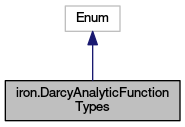OpenCMISS-Iron Python Bindings API Documentation
iron.DarcyAnalyticFunctionTypes Class Reference
Inheritance diagram for iron.DarcyAnalyticFunctionTypes:[legend]
Collaboration diagram for iron.DarcyAnalyticFunctionTypes:[legend]

## Static Public Attributes

int DARCY_EQUATION_TWO_DIM_1 = 1

int DARCY_EQUATION_TWO_DIM_2 = 2

int DARCY_EQUATION_TWO_DIM_3 = 3

int DARCY_EQUATION_THREE_DIM_1 = 4

int DARCY_EQUATION_THREE_DIM_2 = 5

int DARCY_EQUATION_THREE_DIM_3 = 6

int INCOMP_ELAST_DARCY_ANALYTIC_DARCY = 7

## Detailed Description

```The analytic function types for a Darcy equation.
```

Definition at line 19132 of file iron.py.

## Member Data Documentation

 int iron.DarcyAnalyticFunctionTypes.DARCY_EQUATION_THREE_DIM_1 = 4
static

Definition at line 19139 of file iron.py.

 int iron.DarcyAnalyticFunctionTypes.DARCY_EQUATION_THREE_DIM_2 = 5
static

Definition at line 19140 of file iron.py.

 int iron.DarcyAnalyticFunctionTypes.DARCY_EQUATION_THREE_DIM_3 = 6
static

Definition at line 19141 of file iron.py.

 int iron.DarcyAnalyticFunctionTypes.DARCY_EQUATION_TWO_DIM_1 = 1
static

Definition at line 19136 of file iron.py.

 int iron.DarcyAnalyticFunctionTypes.DARCY_EQUATION_TWO_DIM_2 = 2
static

Definition at line 19137 of file iron.py.

 int iron.DarcyAnalyticFunctionTypes.DARCY_EQUATION_TWO_DIM_3 = 3
static

Definition at line 19138 of file iron.py.

 int iron.DarcyAnalyticFunctionTypes.INCOMP_ELAST_DARCY_ANALYTIC_DARCY = 7
static

Definition at line 19142 of file iron.py.